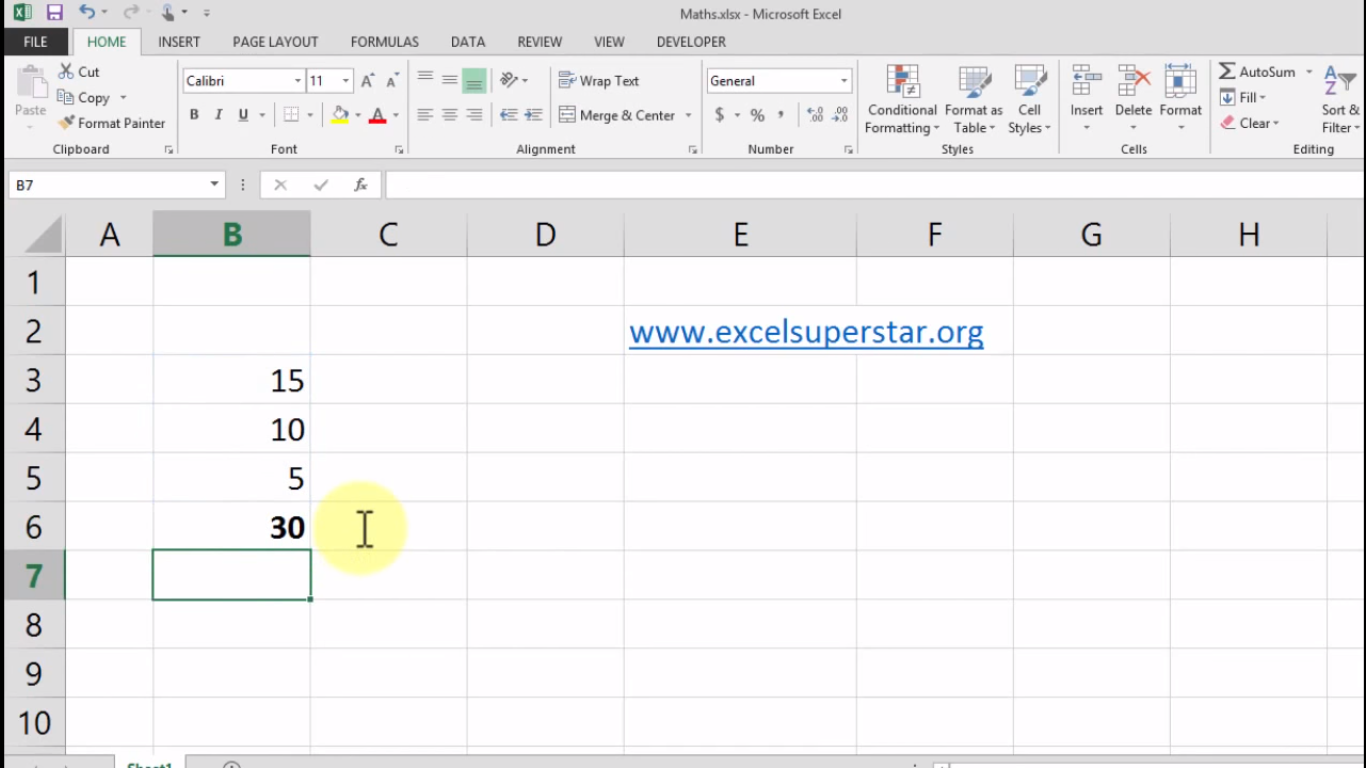# Excel Sum Formula – 3 Easy Ways to DoIn this tutorial we will learn Excel Sum Formula with the help of 3 Ways

1. Method 1 – Primary Method
2. Method 2 – Auto Sum Option
3. Shortcut Key – Alt + =

Method 1 – Primary Method

I have 3 numbers given in Excel, which 15, 10 and 5. Now, if we go through the primary method then what I will do is:

1. Press =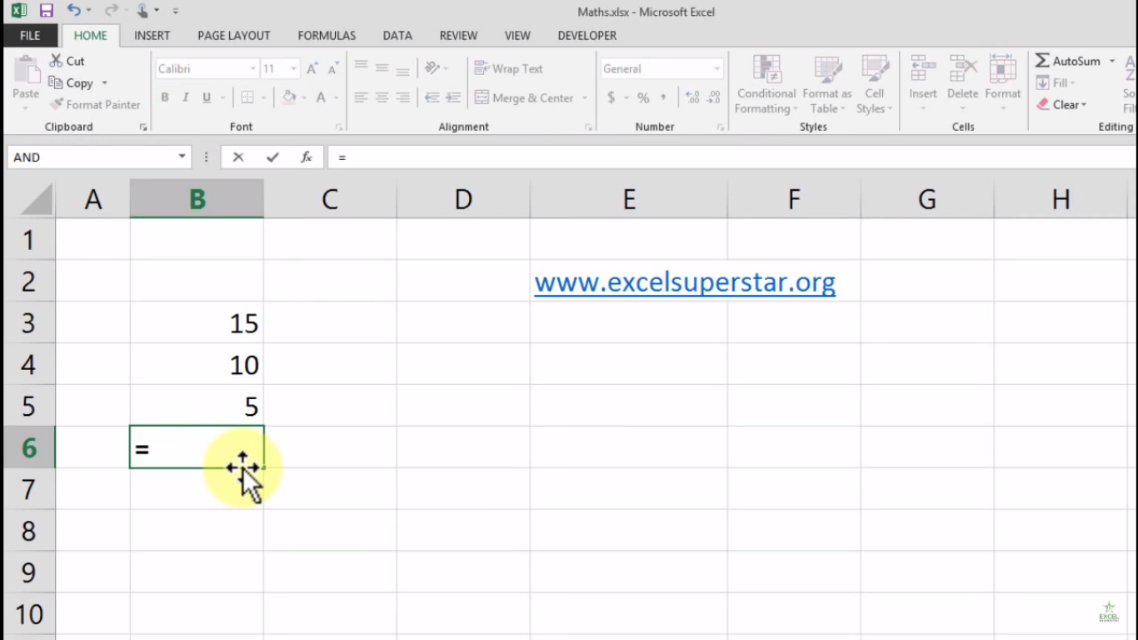2. Then select the number 15 and add it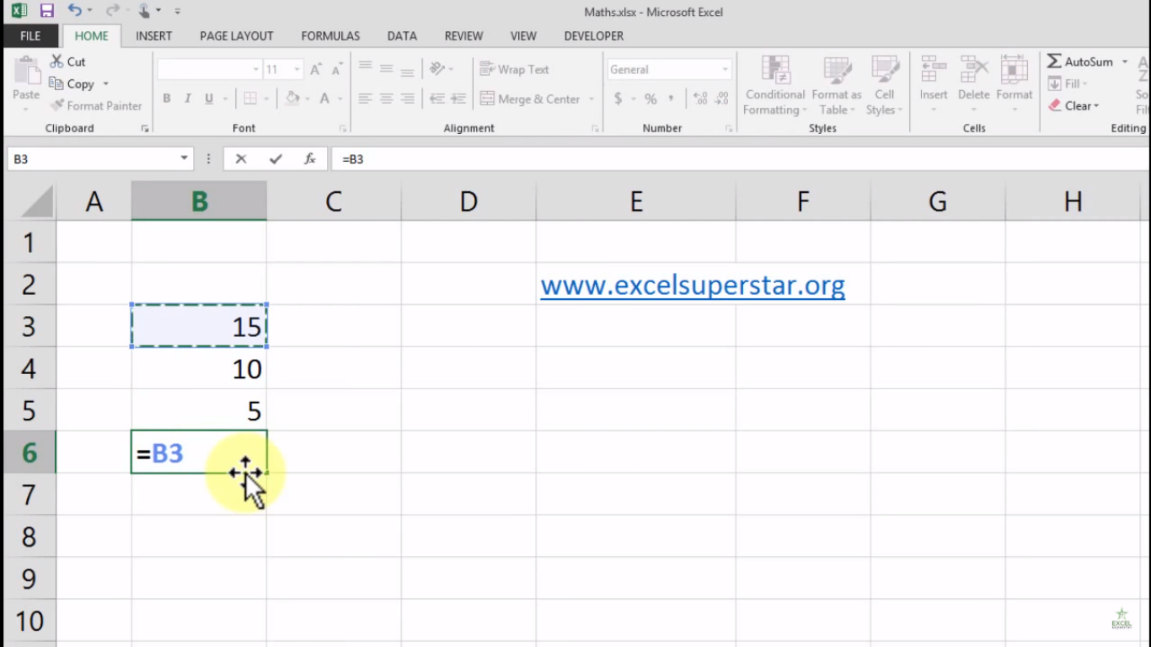3. Then select the number 10 and add it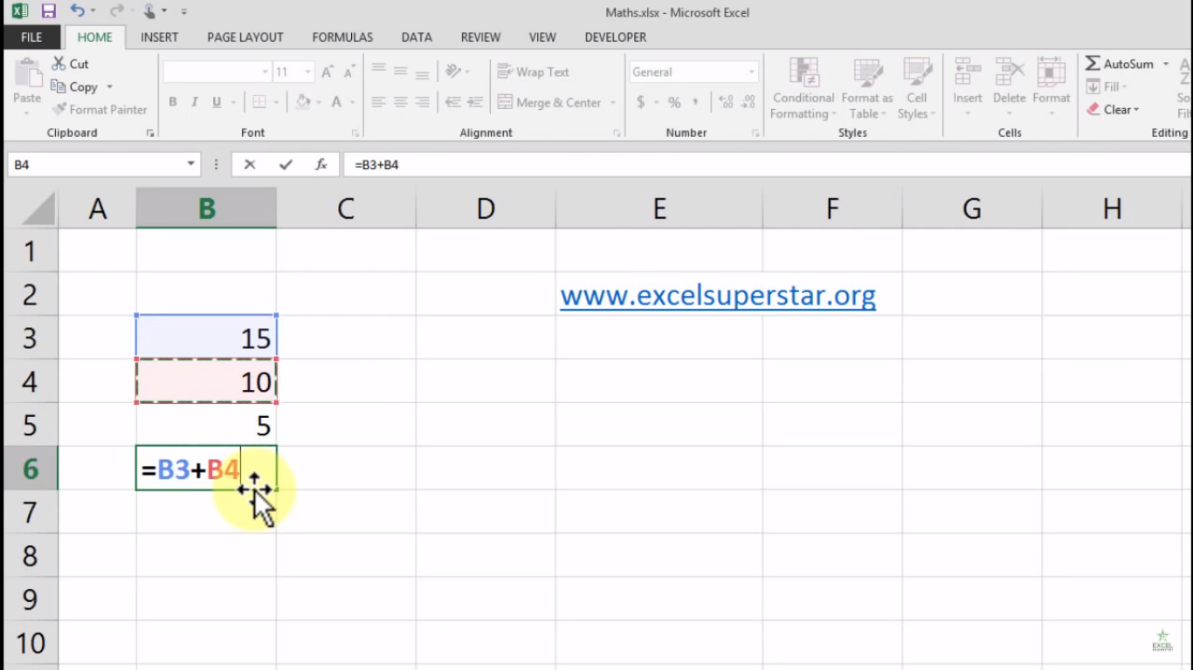4. Again, select the number 5 and add it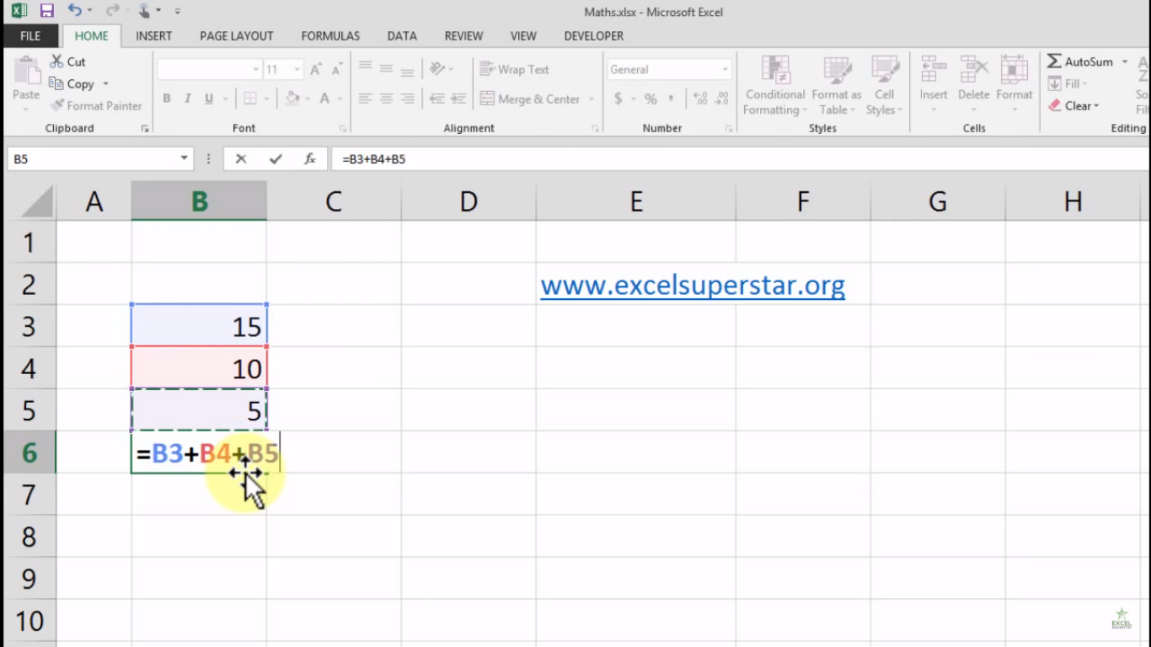5. Lastly, it will add up and will give me the total which is 30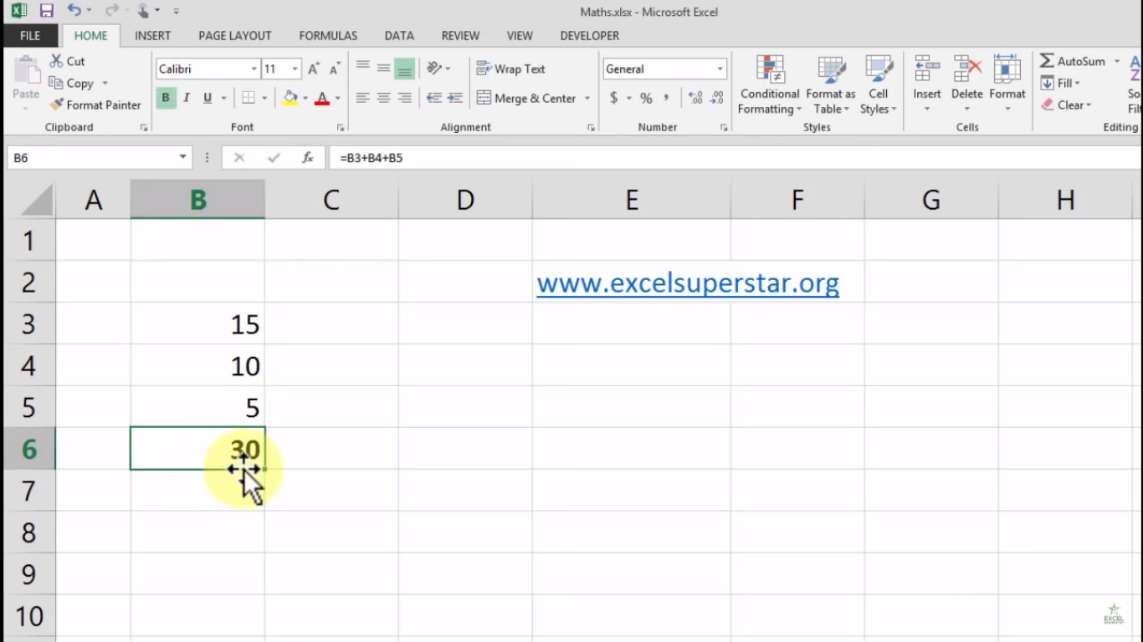Method 2 – Auto Sum Option

1. Choose the cell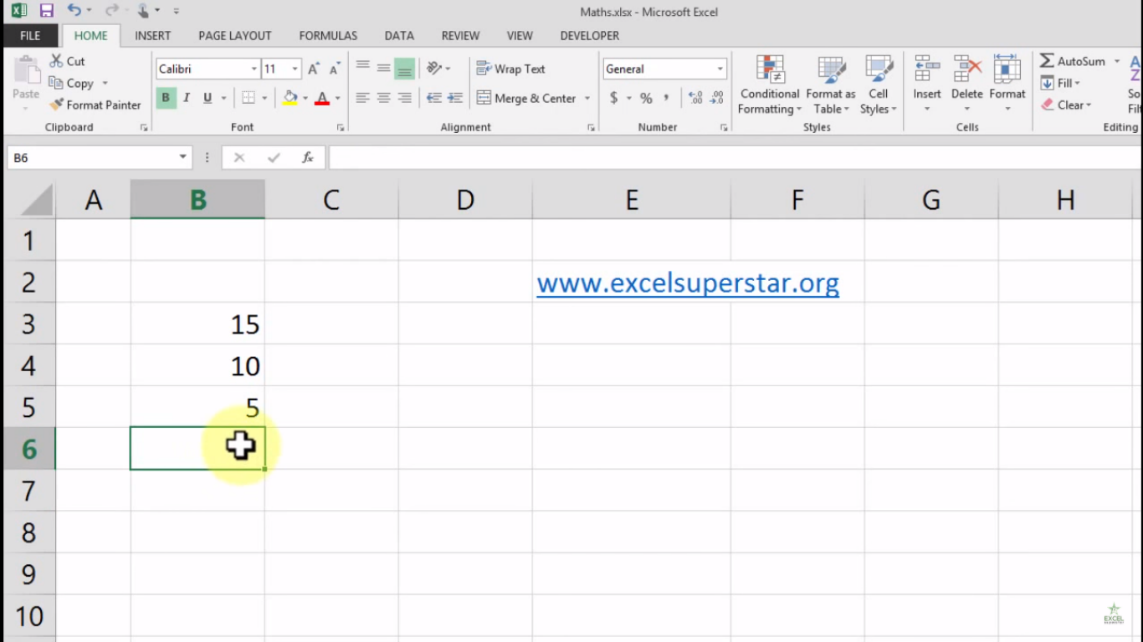2. Go to Home Tab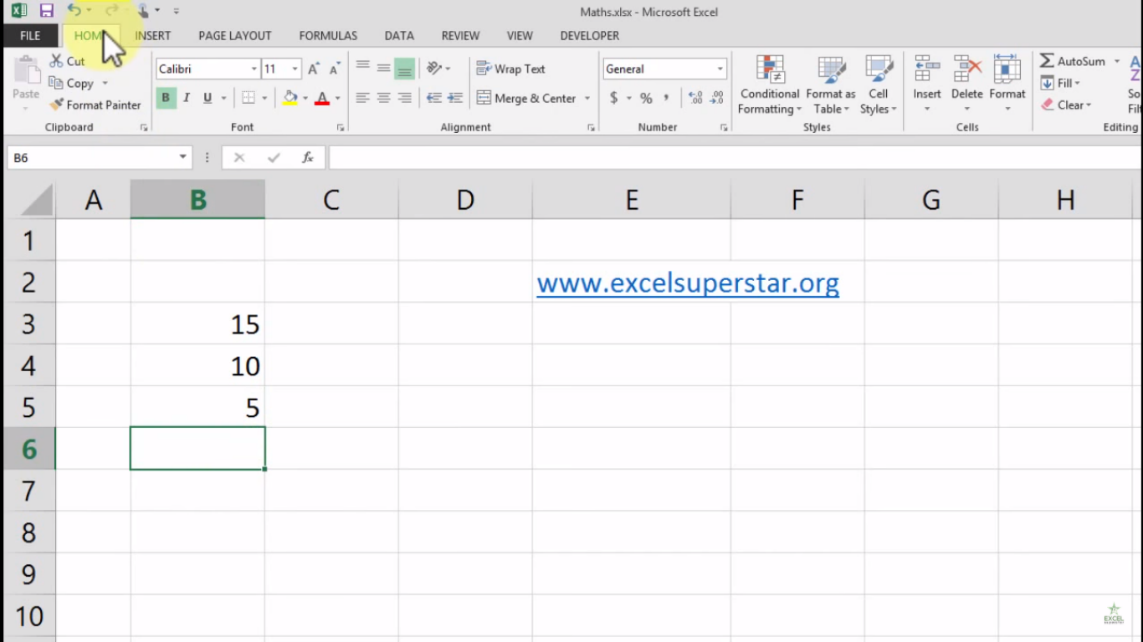3. On the right-hand side there will be an Auto Sum option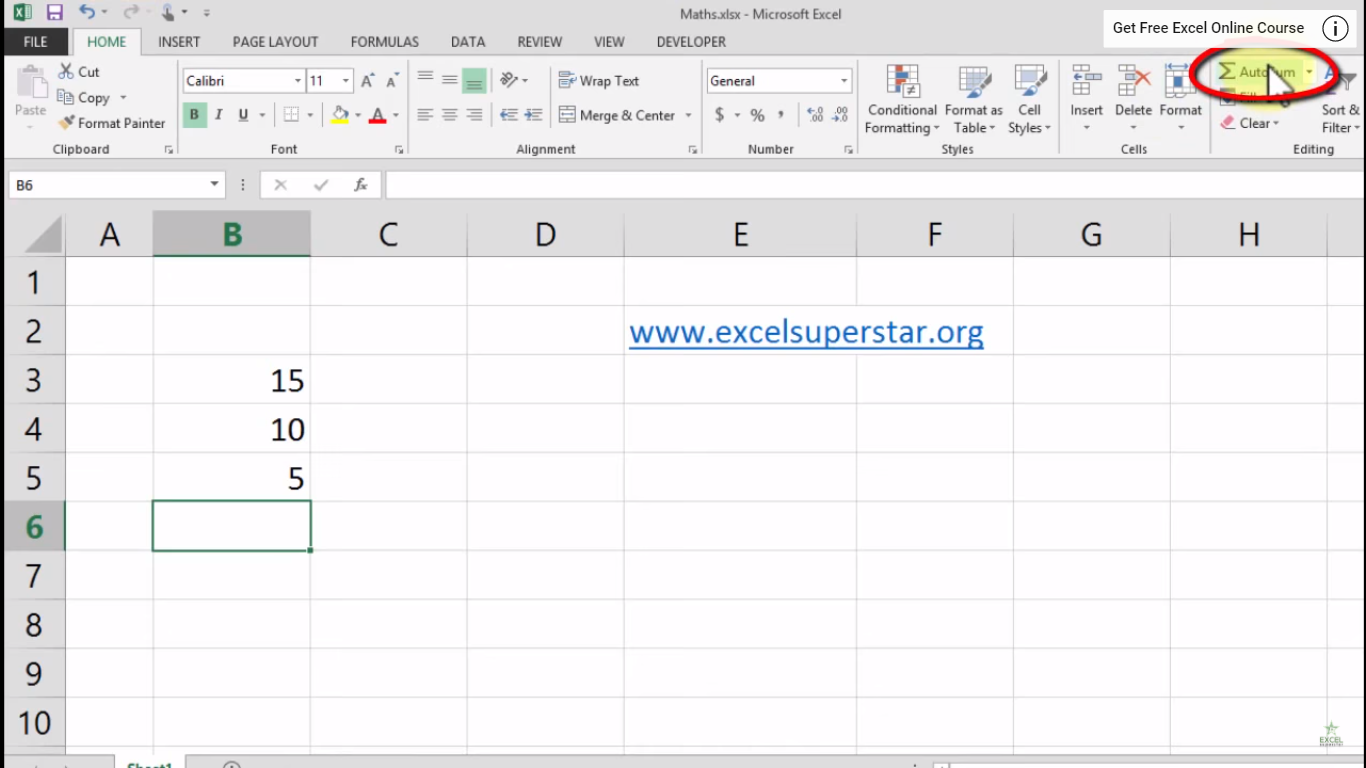4. Click on the Auto sum dropdown

5. There will be various options from there choose Sum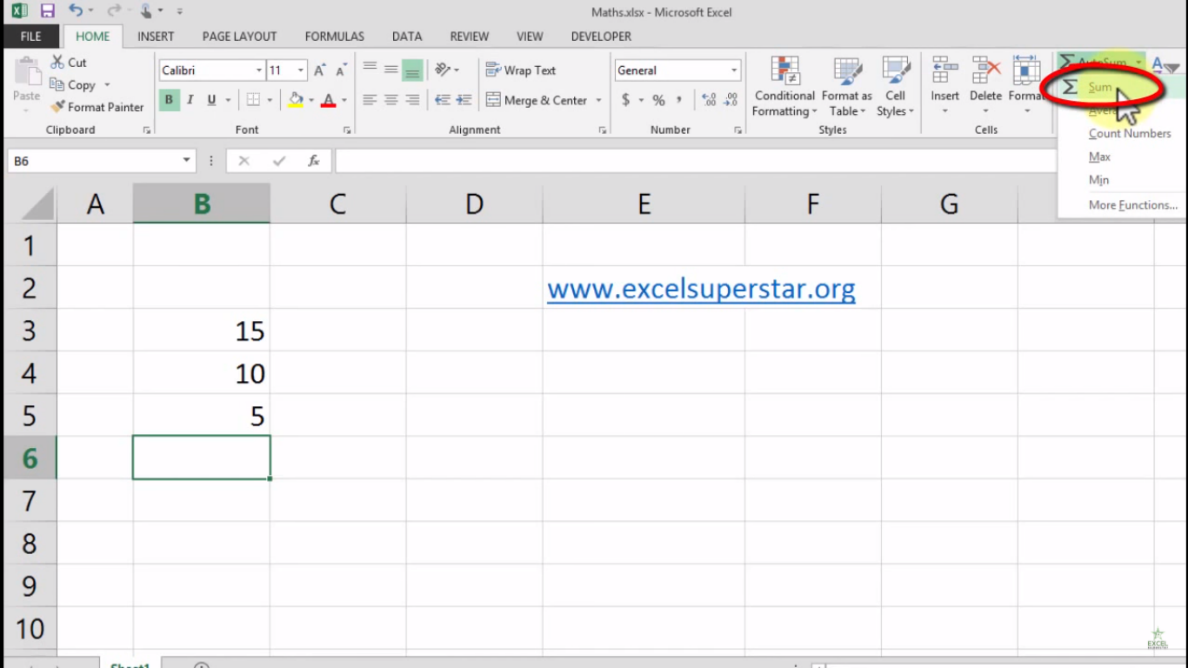6. Now, you will see that there is a function or formula which appeared after clicking on the Sum option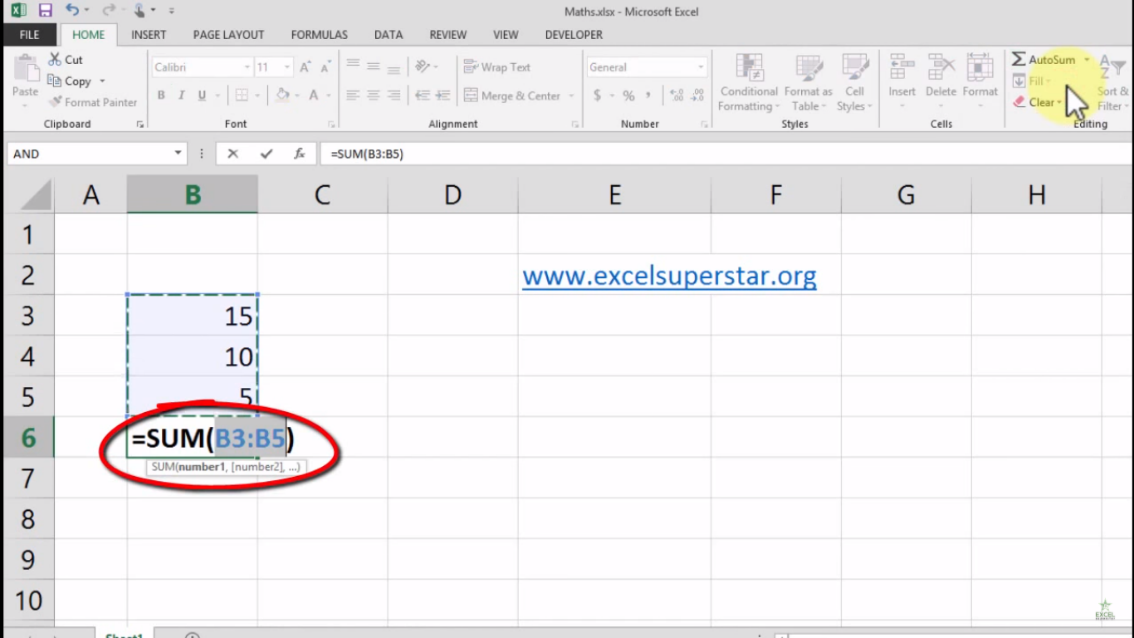Note – A formula is that in which there is a Sum formula, brackets are open and closed in which there are many parameters which can be asked

7. Lastly, Press Enter Key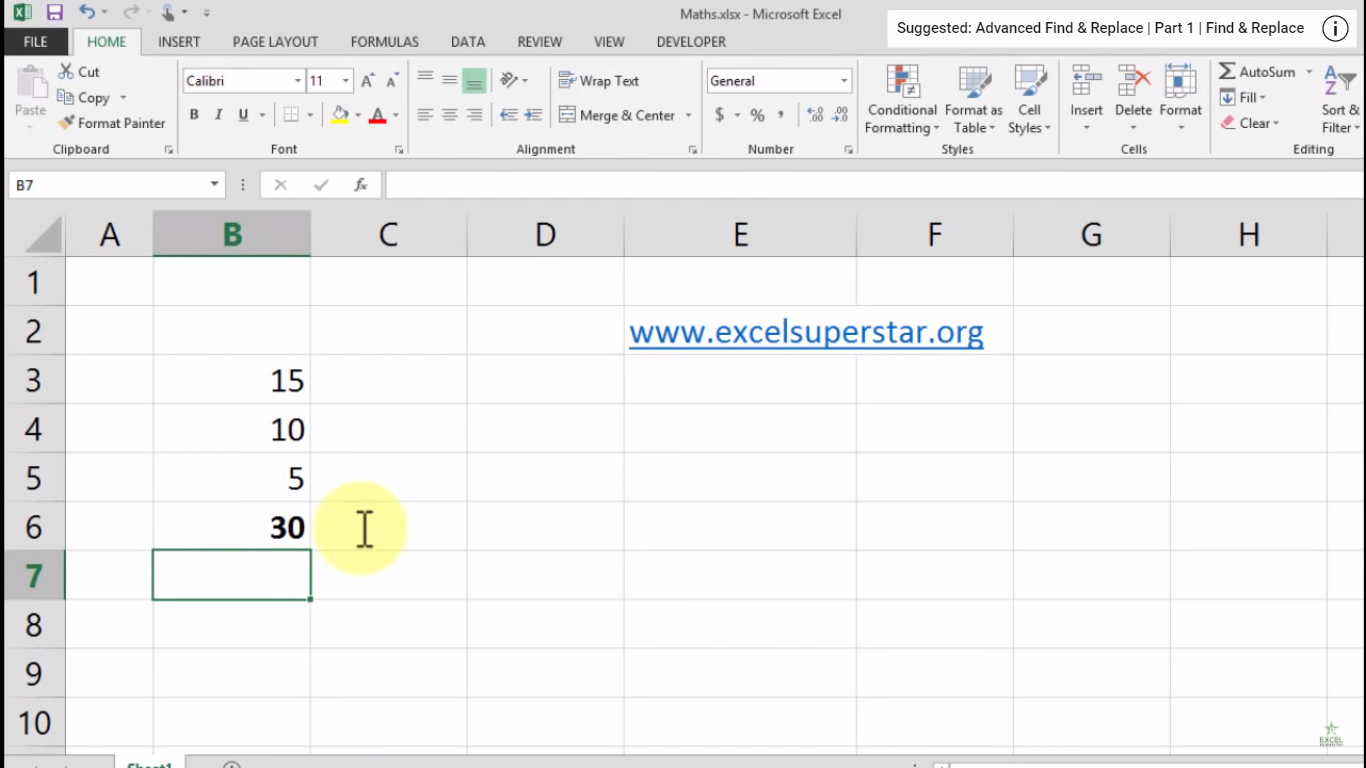3. Shortcut Key Alt + =

1. Press Alt + =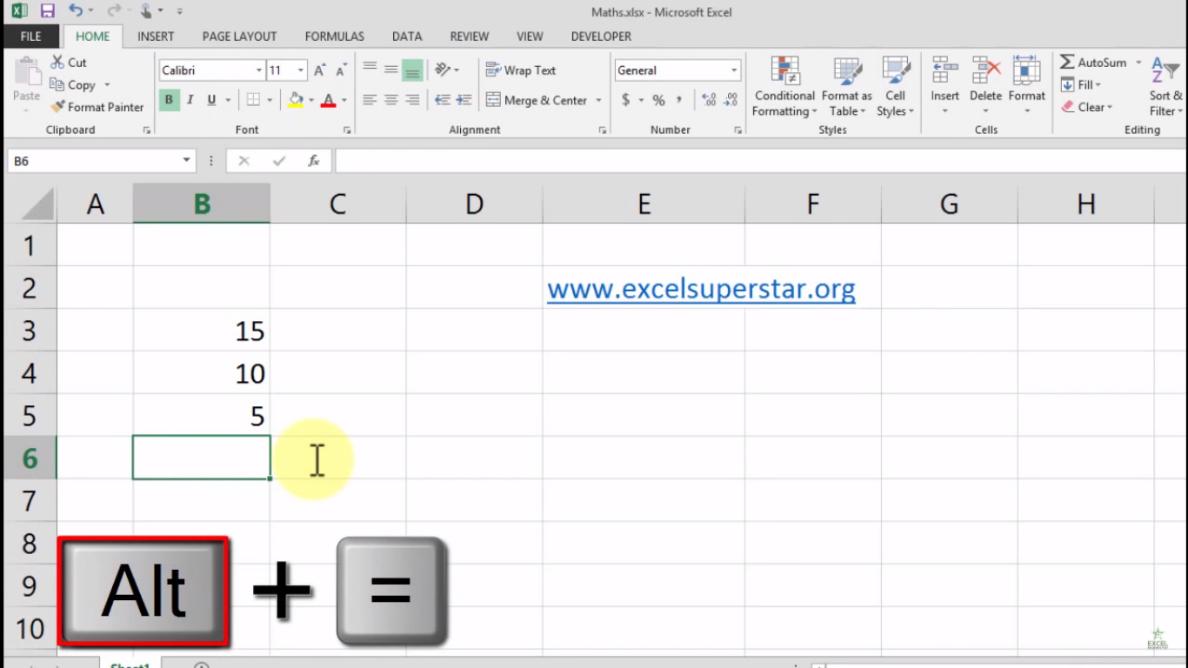2. Press Enter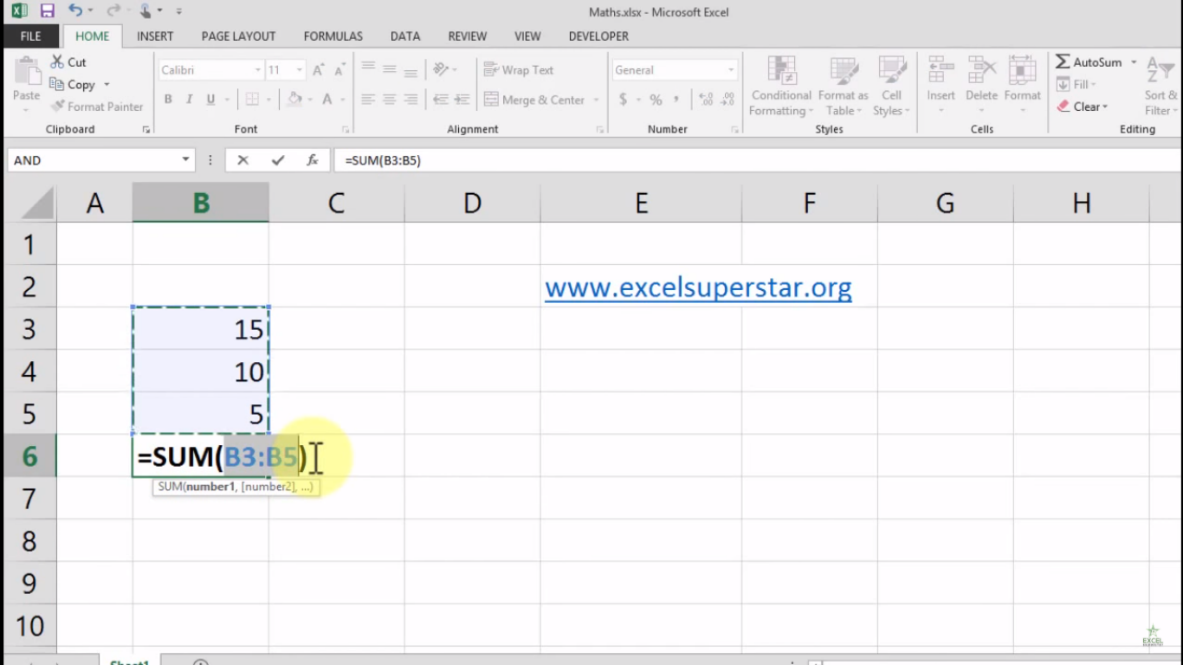3. Lastly, after pressing enter, you will see that your numbers are added easily